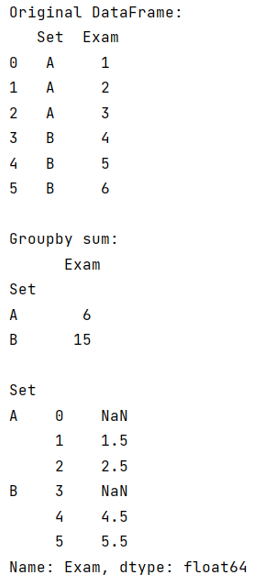# Python Pandas: Rolling functions for GroupBy object

Learn about the rolling functions for GroupBy object in Python Pandas. By Pranit Sharma Last updated : September 17, 2023

Pandas is a special tool that allows us to perform complex manipulations of data effectively and efficiently. Inside pandas, we mostly deal with a dataset in the form of DataFrame. DataFrames are 2-dimensional data structures in pandas. DataFrames consist of rows, columns, and data.

## Problem statement

Given a Pandas DataFrame, we have to roll the groupby sum to work with the grouped objects.

## Rolling functions for GroupBy object

To roll the groupby sum to work with the grouped objects, we will first groupby and sum the Dataframe and then we will use rolling() and mean() methods to roll the grouped objects.

### DataFrame.groupby() Method

The groupby() method is a simple but very useful concept in pandas. By using groupby, we can create a grouping of certain values and perform some operations on those values. It split the object, apply some operations, and then combines them to create a group hence a large amount of data and computations can be performed on these groups.

Let us understand with the help of an example,

## Python program for rolling functions for GroupBy object

```# Importing pandas package
import pandas as pd

# Creating a Dictionary
d = {
'Set':['A','A','A','B','B','B'],
'Exam':[1,2,3,4,5,6]
}

# Creating a DataFrame
df = pd.DataFrame(d)

# Display original DataFrame
print("Original DataFrame:\n",df,"\n")

# Performing groupby sum
result = df.groupby('Set').sum()

# Display result
print("Groupby sum:\n",result,"\n")

# Rolling the grouped objects
print(df.groupby('Set')['Exam'].rolling(2).mean())
```

### Output

The output of the above program is: